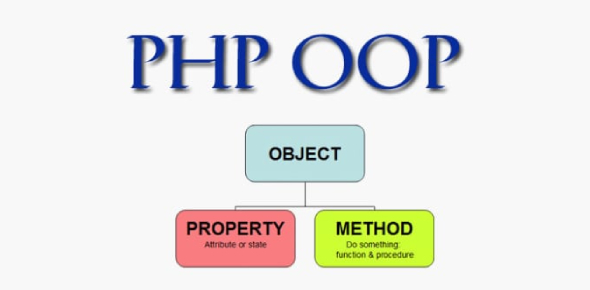# PHP OOP Practice Quiz

38 Questions | Attempts: 3313
ShareSettingsAre you a PHP language expert? What is PHP OOP? Is it hard? Take this PHP practice test and check how easily you can solve basic PHP questions. In this quiz, we’ll be analyzing your knowledge of all the different functions of the widely used programming and web development language PHP! What can you tell us about these functions and how they are used? Give this quiz a try and test your knowledge of this language. Let’s find out!

• 1.
As of PHP 5.3.0, PHP implements a feature which can be used to reference the called class in a context of static inheritance. How is it called?
• A.

Static class bindings

• B.

Static bindings

• C.

Late static bindings

• D.

Late bindings

• E.

Class bindings

• 2.
The above code will:
• A.

Cause error

• B.

Call local version of strlen

• C.

Call global version of strlen

• D.

Work only as of PHP 5.3 and call local version of strlen

• E.

Work only as of PHP 5.3 and call global version of strlen

• 3.
Which function activates the circular reference collector?
• A.

Gc_rc()

• B.

Gc_enable()

• C.

Crc_enable()

• D.

Gc_activate()

• E.

Crc_activate()

• 4.
Does anonymous functions allow creation of functions without specifying their name?
• A.

Yes

• B.

No

• C.

There is nothing like 'anonymouss functions'

• 5.
Mysql_connect() takes parameters:
• A.

(string, string, string, bool, int)

• B.

(string, string)

• C.

(string, string, string, bool)

• D.

(string, string, string, int, bool)

• E.

(string, string, string, bool, bool)

• 6.
What does PHP stand for?
• A.

PHP: Hypertext Processor

• B.

• C.

• D.

Personal Hypertext Processor

• E.

PHP: Personal Hypertext Processor

• 7.
Is it possible to run several versions of PHP at the same time?
• A.

Yes

• B.

No

• C.

Yes, but only commercial editions of PHP

• 8.
PHP scripts are surrounded by delimiters, which?
• A.

• B.

...

• C.

or

• D.

• E.

• 9.
All variables in PHP are prefixed with special symbol, which?
• A.

@

• B.

#

• C.

\$

• D.

\

• E.

%

• 10.
How do You get information from a form that is submitted using the "get" method?
• A.

Request.QueryString;

• B.

Request.Form;

• C.

Get_request();

• D.

\$_GET[];

• E.

Request(GET);

• 11.
In PHP You can use both single quotes (' ') and double quotes (" ") for strings:
• A.

True

• B.

False

• C.

None from above

• 12.
Include files must have file extension *.inc
• A.

True

• B.

False

• 13.
What is the correct way to connect to MySQL database?
• A.

Connect_mysql("localhost");

• B.

Mysql_open("localhost");

• C.

Dbopen("localhost");

• D.

Mysql_connect("localhost");

• E.

Depends on MySQL server version

• 14.
What is the correct way to add 1 to the variable?
• A.

\$variable =+ 1;

• B.

Variable =+1;

• C.

Variable++;

• D.

\$variable++;

• E.

Variable +=1;

• 15.
• A.

*/.../*

• B.

*\...\*

• C.

• D.

#...

• E.

• 16.
Which one of theese variables has illegal name?
• A.

\$myVar

• B.

\$my_Var

• C.

\$my-Var

• D.

All of them

• E.

None of them

• 17.
Can PHP be executed in Command line?
• A.

Yes

• B.

No

• C.

Only on linux

• D.

Only on Windows

• 18.
What Value \$bodytag contains?
• A.

Body text='%black%'

• B.

Body text='black'

• C.

Body text="black"

• D.

None

• 19.
\$data = "foo:*:1023:1000::/home/foo:/bin/sh"; list(\$user, \$pass, \$uid, \$gid, \$gecos, \$home, \$shell) = explode(":", \$data); echo \$user; echo \$pass; ?> What set of value it will give?
• A.

Foo:*1023

• B.

Foo*

• C.

*foo

• D.

Foo

• E.

*

• 20.
\$fruits = array("lemon", "orange", "banana", "apple"); sort(\$fruits); foreach (\$fruits as \$key => \$val) { echo "fruits[" . \$key . "] = " . \$val . "\n"; } ?> The above code will result in:
• A.

Fruits = lemon fruits = orange fruits = banana fruits = apple

• B.

Apple banana lemon orange

• C.

Fruits = apple fruits = banana fruits = lemon fruits = orange

• D.

Fruits = apple fruits = banana fruits = orange fruits = lemon

• E.

None

• 21.
\$Link_ID = odbc_connect("DSN", "user", "pass"); \$Return = odbc_autocommit(\$Link_ID, FALSE); ?> will result in:
• A.

Set autocommit on

• B.

Set autocommit off

• C.

Error in code

• D.

Nothing

• 22.
What's correct result of below code? \$fruits = array("d" => "lemon", "a" => "orange", "b" => "banana", "c" => "apple"); asort(\$fruits); foreach (\$fruits as \$key => \$val) { echo "\$key = \$val\n"; } ?>
• A.

Apple banana lemon a = orange

• B.

C = apple b = banana d = lemon a = orange

• C.

A = apple b = banana c = lemon d = orange

• D.

Apple banana lemon orange

• E.

Apple banana lemon orange

• 23.
If only one array is given in array_merge() as parameter and the array is numerically indexed, the keys get reindexed in a continuous way.
• A.

The above sentense is true

• B.

The above sentense is false

• C.

Described situation will result in error

• 24.
\$array = array('first' => null, 'second' => 4); isset(\$array['first']); ?> Choose the correct answer.
• A.

Isset returns true

• B.

Isset returns false

• C.

Error in code

• 25.
\$email = '[email protected]'; \$domain = strstr(\$email, '@'); echo \$domain; ?> Whats the result of above code?
• A.

Name

• B.
• C.

@example

• D.

@example.com

• E.

Example.com

## Related TopicsBack to top
×

Wait!
Here's an interesting quiz for you.